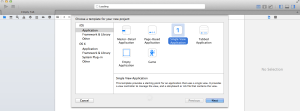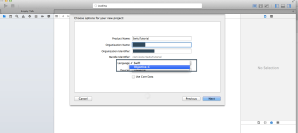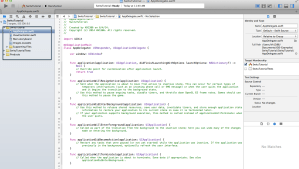# iOS Swift Tutorial – Swift Programming Basics

In iOS Swift Programming tutorial, I have covered how to create Swift Project with Xocde, and Swift language Basics .
Below are the topics covered in this tutorial.

### 1). How to create Swift Project

Swift is introduced in iOS 8, So you need to download XCode 6 to create swift project. Follow the steps
1). In Xcode Menu Go to “File” -> “Project” and select type of your application2).Select Language type as “Swift”.
Xcode 6 supports both Swift and Objective-C languages.2). After creating the project, you can see ViewController and AppDelegeate files extension with “.swift”.3). If you want to create new Swift file, Right click on Project and go to “New File” -> “Swift File”You can import any iOS API using “import”

```import Foundation
import UIKit```

### 2). Variables and Constants

Swift is type safe language.It performs type checks when compiling your code and flags any mismatched types as errors.
This enables you to catch and fix errors as early as possible in the development process
Swift provides its own versions of all fundamental C and Objective-C types,

```Int
Double
Float
Bool
String
Character```

Swift also provides collection types, Array and Dictionary. In Swift, we need not tell the compiler what data type you are going to use.

To create a variable use ‘var‘. var is mutable.
To create constant use ‘let‘. ‘let’ is immutable.

```var var2=20 //20 is integer, so var 2 declared as Integer.
var2 = 20.0 //ERROR. assigning float value  to integer.
var2 = 30 //OK

let const1=10

var var3 = "Ravi"  //"Ravi" is a string, so var3 data types becomes String
var3 = 20 //ERROR: Cannot convert the expression's type '()' to type 'String'

//You can use almost any character you like for constant and variable names, including Unicode characters:
let π = 3.14159
var रवि="Ravi"```

Note: Semicolon is not required at the end of each statement.
But if you want to write two statements in a single line,You need to use semicolon.

`var x=10; println(x)`

### Explicit type annotation

You can provide a type annotation when you declare a constant or variable, to be clear about the kind of values the constant or variable can store.
Write a type annotation by placing a colon after the constant or variable name, followed by a space, followed by the name of the type to use.

```var var6:(Int) = 30
var var7:(String) = "Ravi"
var var8:(Bool) = true```

Conversion between integer and floating point number made this way.

```var var10:(Int) = Int(10.01)  //Double is converted to Int

var var11:(Double) = Double(21)  //Int is converted to Double```

### 2.1) Swift Strings

Below are the different examples on Swift strings

```//1.Create empty string
var str = "" //emtpy string literal
var str1 = String() //empty string

//2.Check if string is empty
if str.isEmpty
{

}

//3.Check length of string
countElements(str)

//4.String Concatenation

var str2 = "Hello"
var str3 = "World"
var str4 = str2+" "+str3

//Iterate through String
var str2 = "Hello"
for char in str2
{
println(char) //each character
}

//5.Iterate through UTF-8

var name = "रवि"; //UTF8-Code 224 164 176 224 164 181 224 164
for code in name.utf8
{
println(code); // each UTF8 code
}

//6.Formatting String
var int1 = 33
var str5 = "Ravi age \(int1)"  //Ravi age 33

//7.Comparing Strings
if str2 == str4
{
println("Equal")
}```

### 2.2) Swift Arrays

Below are the different examples on Swift Arrays.

```//1.Creating an empty array
var empty = [];

//2.Create an initialized array
var names=["Ravi","Haya","Rama"]
var names2:String[] = ["Ravi","Haya","Rama"] //with explicit type convention

var numbers = [1,2,4,5,6,7,8]

//3.Get the count of an array
println(numbers.count)

//4. Iterate through each element in array
//Method 1
for (var i=0;i<numbers.count;i++)
{
println(numbers[i])
}

//Method 2
for num in numbers
{
println(num)
}

//Method 3
for (index,value)  in enumerate(numbers)
{
println("Index \(index): value  \(value)")
}

//5.Create sub array with index range
var subnumbers = numbers[2..4] //4,5
subnumbers = numbers[2...4] //4,5,6

numbers.append(10);

//7.To insert at specific index
numbers.insert(12,atIndex: 5)

//8.Remove element at index
var removed = numbers.removeAtIndex(0)

//9.Remve last element
var removed = numbers.removeLast()```

### 2.2).Dictionary

Below are the different examples on Swift Dictionary

```//1.Create an empty dictionary
var emptyDict = Dictionary<Int,String>();

//2.Create an dictionary with key value pairs
var dict1:Dictionary<Int,String> = [ 1:"One",2:"TwO",3:"Three"];
var dict2 = ["1":"One","2":"Two","3":"Three"];

//3.Iterate through dictionary
for(key,value) in dict1
{
println("key: \(key) value:\(value)")
}

//4.Dictionary element are accessed using subscript.
print(dict["1"]);
dict["1"]="Modifed";

//5.Update an element
dict1.updateValue("Modified",forKey:"2");

//6.Remove an element
dict1["1"] = nil;
//or
dict1.removeValueForKey("1");```

### 2.3) Swift Tuples

Swift provides python like tuple datatype.Tuples group multiple values into a single compound value.

```//1.Creating tuple
let digits = (1,"One")  //digits is type of (Int,String)

let(num,name) = digits
println("Name \(num) Name:\(name)")

//3.Create tuple with custom keys
let digits2 = (num:2,name:"Two")
println("Name \(digits2.num) Name:\(digits2.name)")```

### 3) Printing Variables and Constants

you can use println() function to print variables.
To print the variable you need to wrap the variable name in parenthesis: \(VARIABLE)

```//1. Print string
println("Ravi")

//2. Print variables
var x=10
var  y=10
println("x: \(x)  y:\(y)")

//3. using NSLog
NSLog("Ravi")
NSLog("x:%d",x)```

### 4).Conditional Statements

Swift provides all conditional statements Objective-C provides. Swift conditional statements are similar to Objective-C, parenthesis are not mandatory. Swift conditional statements are similar to Objective-C, parenthesis are not mandatory.switch statement doesn’t need break statement, it does not fall through the bottom of each case.

```//1.If else statement
var status = true
if status
{
println("YES")
}
else
{
println("NO")
}

//2.Switch Statement
let char = "a"

switch char
{
case "a","b","c":
println("First Three")
case "x","y","z":
println("Last Three")
case "o":
println("OOO")
default:
println("NONE");
}

//3. Switch with Range matching
let num = 1
switch num
{
case 0...99:
println("Below 100");
case 100...999:
println("Between 100 & 999")
default:
print("Out of range");

}```

### 5) Control Statements( Loops )

Swift supports for-in, for, while,do-while control statements. Below are the examples.

```//1.for-in example

var str="Ravi";
for char in str
{
println(char);
}

var arr = [1,2,4,5]
for value in arr
{
println(value);
}

//.. defines range from 1 to 10, does not include 10
for val in 1..10
{
println(val);
}

//.. defines range from 1 to 10,includes 10
for val in 1...10
{
println(val);
}

//2. For loop
for var i = 0;i<10;i++
{
println(i);
}

//3. While loop
var i=0
while i < 10
{
println(i)
i++
}

//4. do-while loop
var i=0

do
{
i++
println(i)
}while i < 10```